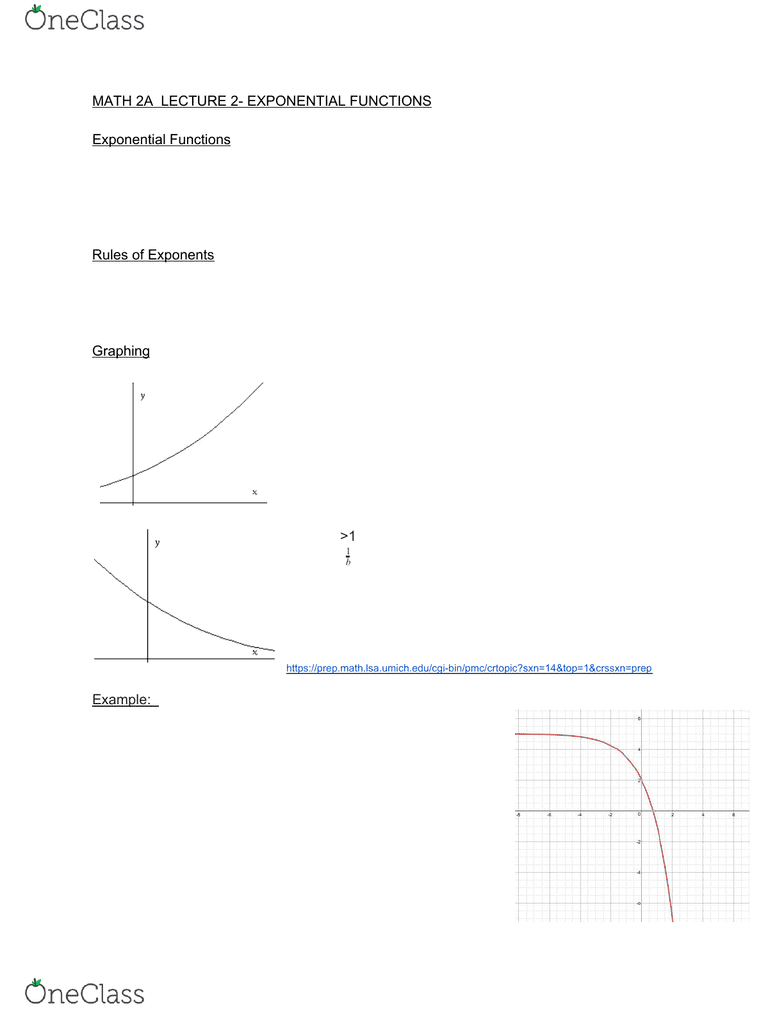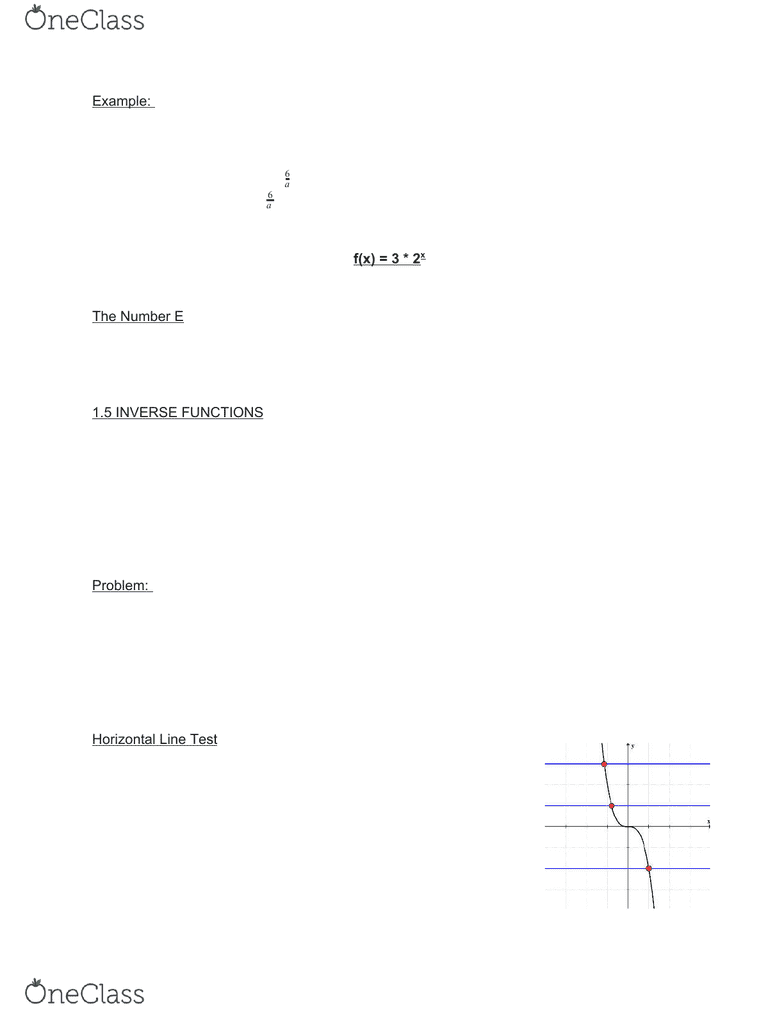Study Guides (390,000)
US (220,000)
UC-Irvine (3,000)
MATH (600)
MATH 2A (60)
Liu, W (1)
Study Guide

# MATH 2A Study Guide - Fall 2018, Comprehensive Midterm Notes - Graph Of A Function, Integer, TranscendentalsPremium

Department
Mathematics
Course Code
MATH 2A
Professor
Liu, W
Study Guide
Midterm

This preview shows pages 1-3. to view the full 22 pages of the document.University of California - Irvine
MATH 2A
Single-Variable Calculus
Midterm
Fall 2018
Prof. Liu, W

Only pages 1-3 are available for preview. Some parts have been intentionally blurred.

Subscribers OnlyOnly pages 1-3 are available for preview. Some parts have been intentionally blurred.

Subscribers OnlyMATH 2A LECTURE 2- EXPONENTIAL FUNCTIONS
Exponential Functions
f(x) = ax
A is called the base and a > 0
x=n. N is a positive integer.
● An = a * a * a
Rules of Exponents
1) Ax+y = Ax * Ay : Adding exponents is the same as multiplying the exponential functions
2) Ax-y = Ax / Ay : Subtracting exponents is the same as dividing the exponential functions
3) Axy = (Ax)y
Graphing
- a>1
- Increasing
- Domain: all real numbers (- , )
- Ax is not equal to 0 and is not negative
- a>1
- (( )x = bx)
b
1
- The base graph is being reflected on the y-axis.
- Decreasing
- Domain: all real numbers (- , )
- Range: (0,∞)
Example: Sketch the graph of y = 5 -3 * 2x
2) Shift the graph UP THREE spaces to account for
the times 3.
3) REFLECT the graph to account for the negative
4) Shift the graph UP FIVE spaces to account for the
5.
5) The graph should look like this in the end.
find more resources at oneclass.com
find more resources at oneclass.com

Unlock to view full version

Subscribers Only

Only pages 1-3 are available for preview. Some parts have been intentionally blurred.

Subscribers OnlyExample: Find the exponential function whose graph passes through (1,6) and (3,24)
f(1) = 6 f(3) =24
y = f(x) = cax
c*a = 6 c= a
6
c* a3 = 24 * a3 = 24
6
a
6a2 = 24
A2 = 4
a=2, c = 3 so f(x) = 3 * 2x
The Number E
Slopes of tangent lines at (0,1) to exponential graph will cover all possible values.
There is an exponential where the slope is 1. This will have base e (ex).
1.5 INVERSE FUNCTIONS
Function from A → B...create a function g
Essentially, you are switching x and y in order to make an inverse
Ex:
f(1) = 3 and g(3)= 1
f(x) = y → g(y)= x
Problem: f(1,2,3) → (1,2)
f(1) = 1 g(1,2) → (1,2,3)
f(2) = 2 g(1) = 1
f(3) = 2 f-1 (2) → (2,3)
If f has an inverse, f must only take each value once. This is called a one to one function.
Horizontal Line Test
For a one to one function, every horizontal line must touch the
graph of f at least once.
https://www.chilimathwords.com/math-words-starting-with-letter-h/horizontal-line-test/
find more resources at oneclass.com
find more resources at oneclass.com